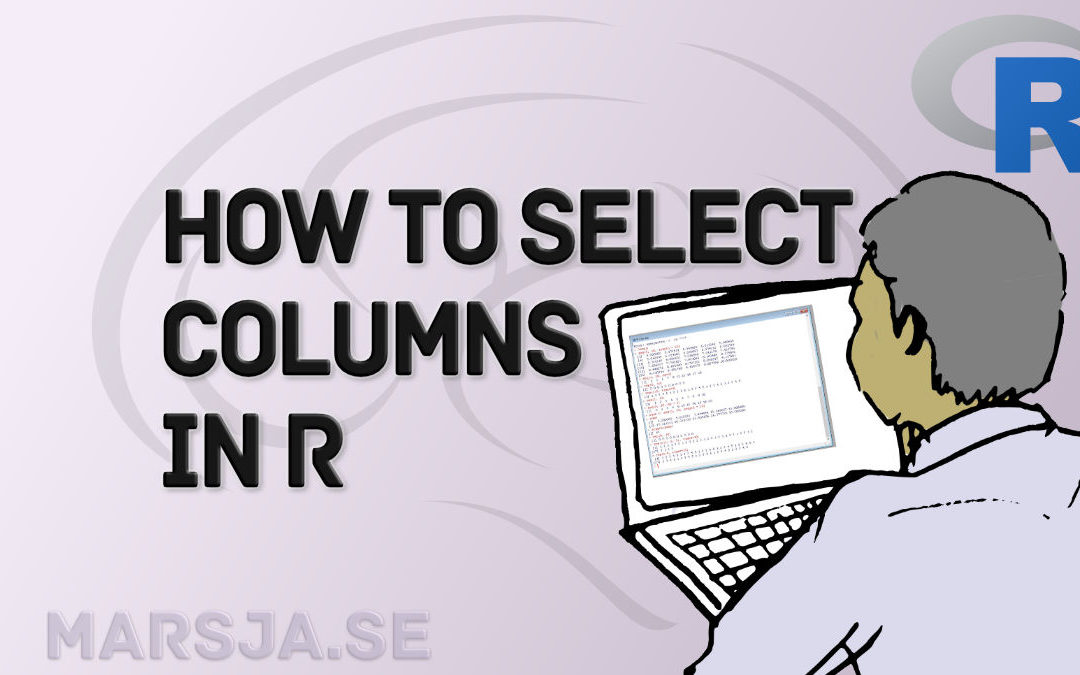## Select Columns in R by Name, Index, Letters, & Certain Words with dplyr

In this R tutorial, you will learn how to select columns in a dataframe. First, we will use base R, in a number of examples, to choose certain columns. Second, we will use dplyr to get columns from the dataframe. Outline In the first section, we are going to have a...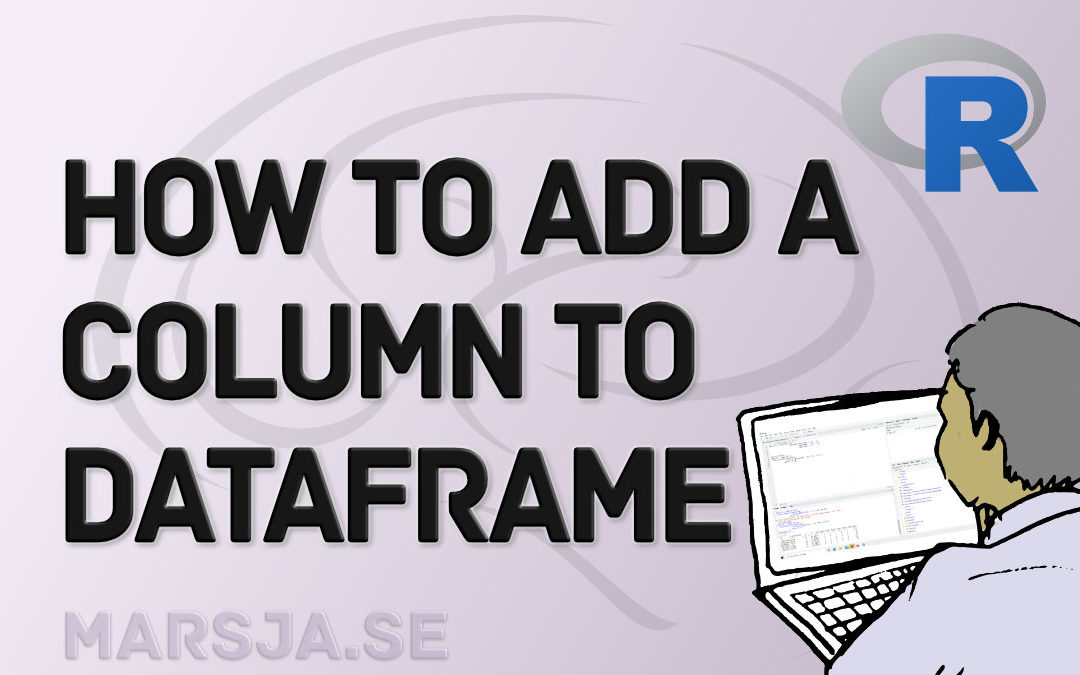## How to Add a Column to a Dataframe in R with tibble & dplyr

In this brief tutorial, you will learn how to add a column to a dataframe in R. More specifically, you will learn 1) to add a column using base R (i.e., by using the \$-operator and brackets, 2) add a column using the add_column() function (i.e., from tibble), 3) add...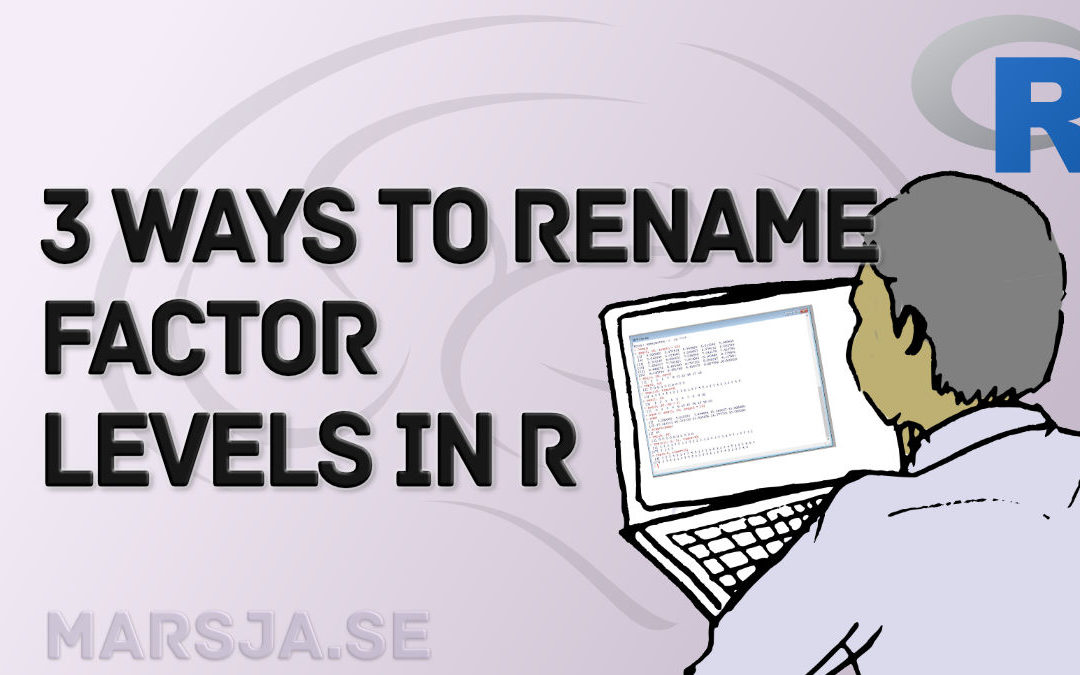## How to Rename Factor Levels in R using levels() and dplyr

In this tutorial, you will learn how to rename factor levels in R. First, we will use the base functions that are available in R, and then we will use dplyr.  To rename factor levels using levels() we can assign a character vector with the new names. If we want...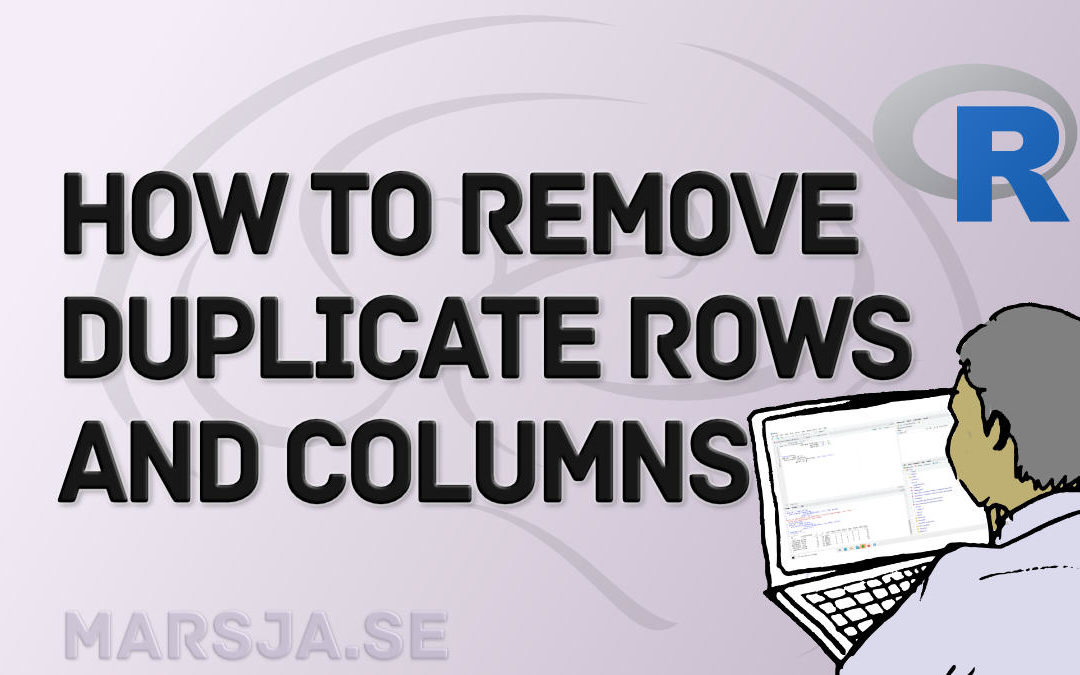## How to Remove Duplicates in R – Rows and Columns (dplyr)

In this R tutorial, you will learn how to remove duplicates from the data frame. First, you will learn how to delete duplicated rows and, second, you will remove columns. Specifically, we will have a look at how to remove duplicate records from the data frame using 1)...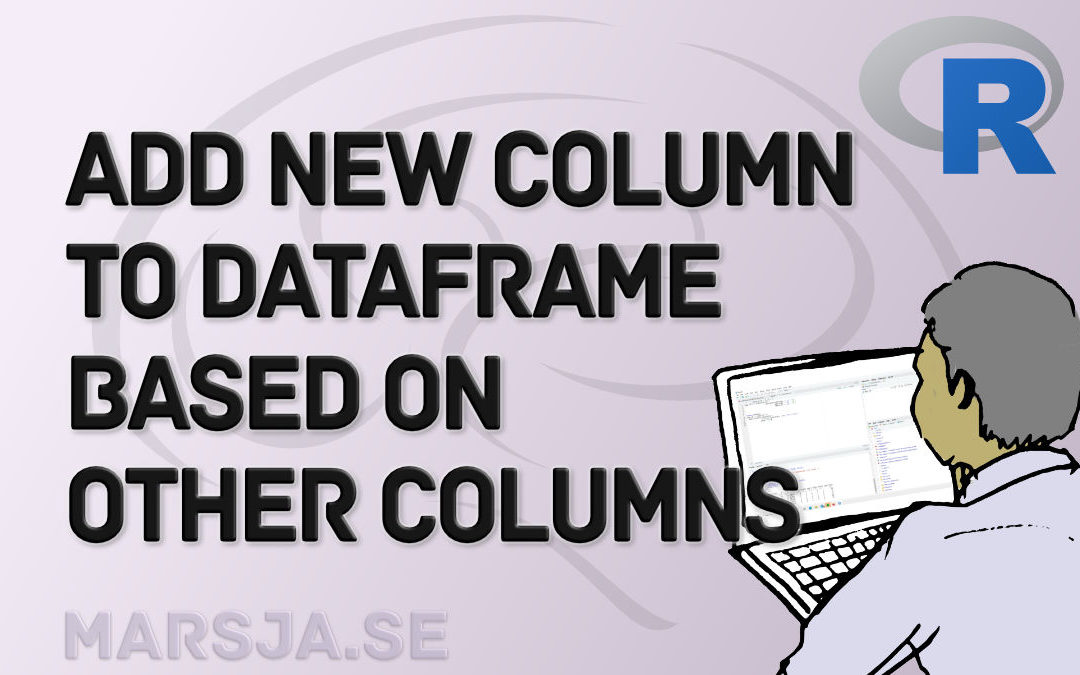## R: Add a Column to Dataframe Based on Other Columns with dplyr

In this R tutorial, you are going to learn how to add a column to a dataframe based on values in other columns. Specifically, you will learn to create a new column using the mutate() function from the package dplyr, along with some other useful functions. Finally, we...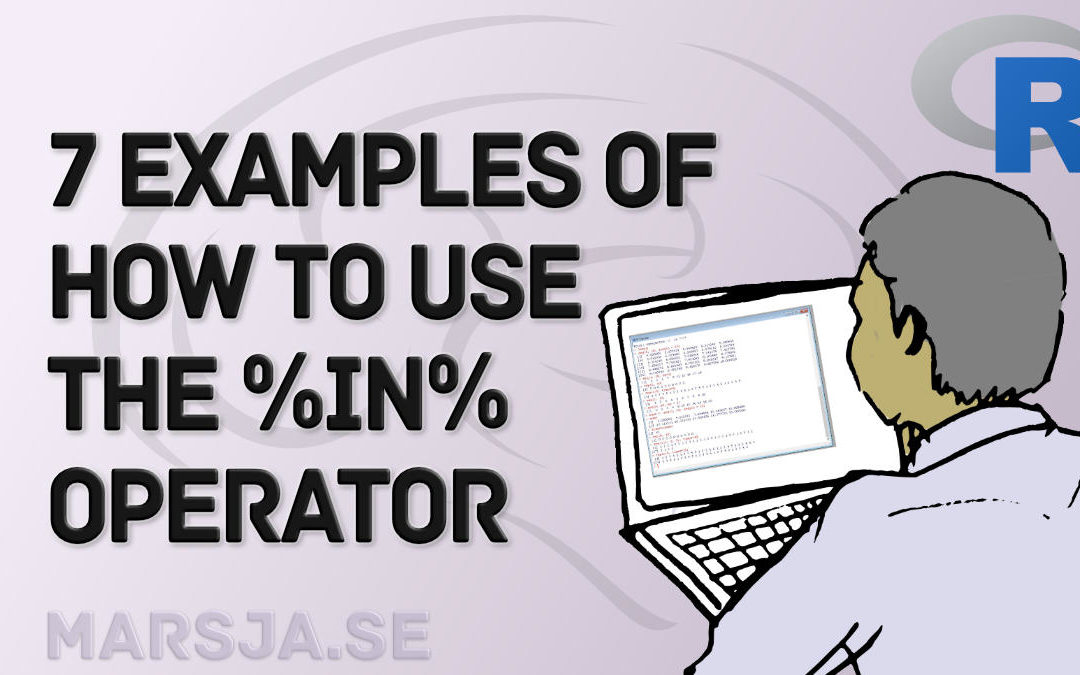## How to use %in% in R: 7 Example Uses of the Operator

In this tutorial, you will learn by examples how to use the %in% in R. Specifically, you will learn 7 different uses of this great operator. Outline Here’s the outline of this post, described a bit more detailed than the table of contents. First, we start out with a...